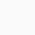Course

# Mathematical Optimization for Engineers

👤 Join WhatsApp Learners
New here?
Deal Score0

Learn the mathematical and computational basics for applying optimization successfully. Master the different formulations and the important concepts behind their solution methods. Learn to implement and solve optimization problems in Python through practical exercises.

### Join Email Learners

Follow the guide on landing page

Today, for almost every product on the market and almost every service offered, some form of optimization has played a role in their design.

However, optimization is not a button-press technology. To apply it successfully, one needs expertise in formulating the problem, selecting and tuning the solution algorithm, and finally, checking the results. We have designed this course to make you such an expert.

This course is useful to students of all engineering fields. The mathematical and computational concepts that you will learn here have applications in machine learning, operations research, signal and image processing, control, robotics, and design to name a few.

We will start with the standard unconstrained problems, linear problems, and general nonlinear constrained problems. We will then move to more specialized topics including mixed-integer problems; global optimization for non-convex problems; optimal control problems; machine learning for optimization and optimization under uncertainty. Students will learn to implement and solve optimization problems in Python through practical exercises.

• Mathematical definitions of the objective function, degrees of freedom, constraints and optimal solution
• Mathematical as well as an intuitive understanding of optimality conditions
• Different optimization formulations (unconstrained v/s constrained; linear v/s nonlinear; mixed-integer v/s continuous; time-continuous or dynamic; optimization under uncertainty)
• Fundamentals of the solution methods for each of these formulations
• Optimization with machine learning embedded
• Hands-on training in implementing and solving optimization problems in Python, as exercises

Week 1: Introduction and math review

• Mathematical definitions of the objective function, degrees of freedom, constraints and optimal solution with real-world examples
• Review of some mathematical basics needed to take us through the course

Week 2: Unconstrained optimization

• Basics of iterative descent: step direction and step length
• Common algorithms like steepest descent, Newton’s method and its variants and trust-region methods.

Week 3: Linear optimization

• KKT conditions of optimality for constrained problems
• Simplex method
• Interior point methods

Week 4: Nonlinear optimization

• Penalty, log-barrier and SQP methods

Mixed-integer optimization

• Branch and bound method for mixed-integer linear problems

Week 5: Global optimization

• Branch and bound method for nonlinear non-convex problems
• Constructing relaxations
• Different formulations and their numerical performance
• Stochastic methods, genetic algorithm,s and derivative-free methods

Week 6: Dynamic optimization

• Full discretization, single-shooting and multi-shooting methods
• Nonlinear model predictive control

Week 7: Machine learning for optimization

• Mechanistic, data-driven and hybrid modelling
• Basics of training machine learning models
• Optimization with machine learning embedded

Week 8: Optimization under uncertainty

• Parametric optimization
• Two stage stochastic problems
• Robust optimization via semi-infinite problems
Mathematical Optimization for Engineers
0DISCLAIMER: Courses on Future Syllabus are free but subject to return to their original prices on host platforms upon coupon expiration. Enrol while they are free.#### Silas Bamigbola

Certified Computer Engineer & Author, 'Lost Boys'. I believe in the influence of right information. Join me on Twitter.

Stay activeRegister New Account